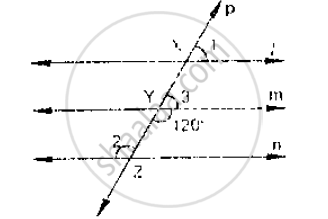# In the Below Fig, L, M and N Are Parallel Lines Intersected by Transversal P at X, Y and Z Respectively. Find ∠1, ∠2 and ∠3. - Mathematics

In the below fig, l, m and n are parallel lines intersected by transversal p at X, Y and Z
respectively. Find ∠1, ∠2 and ∠3.#### Solution

From the given figure:

∠3 + ∠m YZ = 180°          [Linear pair]

⇒ ∠3 = 180° -120°

⇒∠3 = 60°

Now line l parallel to m

∠1 = ∠3              [Corresponding angles]

⇒ ∠1 = 60°

Also m || n

⇒ ∠2 =120°                   [Alternative interior angle]

∴ ∠1 = ∠3 = 60°

∠2 = 120°

Is there an error in this question or solution?
Chapter 10: Lines and Angles - Exercise 10.4 [Page 46]

#### APPEARS IN

RD Sharma Mathematics for Class 9
Chapter 10 Lines and Angles
Exercise 10.4 | Q 2 | Page 46

Share